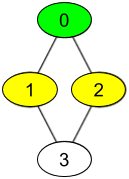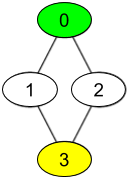# GeetCode Hub

There are `n` people, each person has a unique id between `0` and `n-1`. Given the arrays `watchedVideos` and `friends`, where `watchedVideos[i]` and `friends[i]` contain the list of watched videos and the list of friends respectively for the person with `id = i`.

Level 1 of videos are all watched videos by your friends, level 2 of videos are all watched videos by the friends of your friends and so on. In general, the level `k` of videos are all watched videos by people with the shortest path exactly equal to `k` with you. Given your `id` and the `level` of videos, return the list of videos ordered by their frequencies (increasing). For videos with the same frequency order them alphabetically from least to greatest.

Example 1:```Input: watchedVideos = [["A","B"],["C"],["B","C"],["D"]], friends = [[1,2],[0,3],[0,3],[1,2]], id = 0, level = 1
Output: ["B","C"]
Explanation:
You have id = 0 (green color in the figure) and your friends are (yellow color in the figure):
Person with id = 1 -> watchedVideos = ["C"]
Person with id = 2 -> watchedVideos = ["B","C"]
The frequencies of watchedVideos by your friends are:
B -> 1
C -> 2
```

Example 2:```Input: watchedVideos = [["A","B"],["C"],["B","C"],["D"]], friends = [[1,2],[0,3],[0,3],[1,2]], id = 0, level = 2
Output: ["D"]
Explanation:
You have id = 0 (green color in the figure) and the only friend of your friends is the person with id = 3 (yellow color in the figure).
```

Constraints:

• `n == watchedVideos.length == friends.length`
• `2 <= n <= 100`
• `1 <= watchedVideos[i].length <= 100`
• `1 <= watchedVideos[i][j].length <= 8`
• `0 <= friends[i].length < n`
• `0 <= friends[i][j] < n`
• `0 <= id < n`
• `1 <= level < n`
• if `friends[i]` contains `j`, then `friends[j]` contains `i`

class Solution { public List<String> watchedVideosByFriends(List<List<String>> watchedVideos, int[][] friends, int id, int level) { } }